# Electricity slides

4. Aug 2010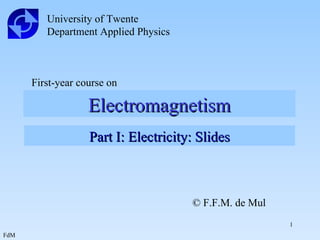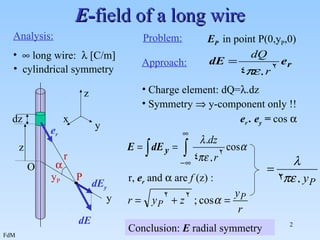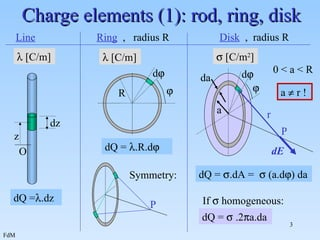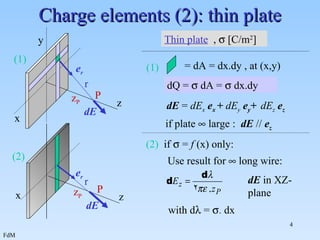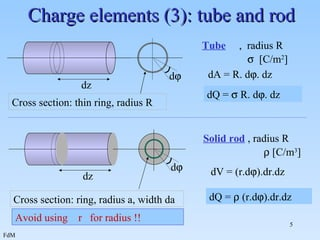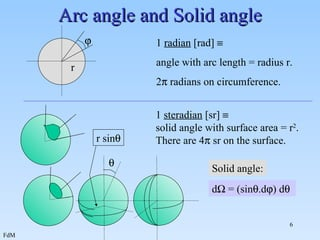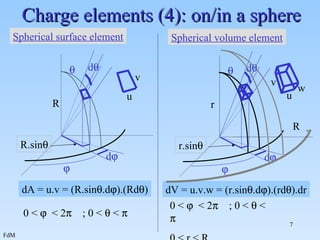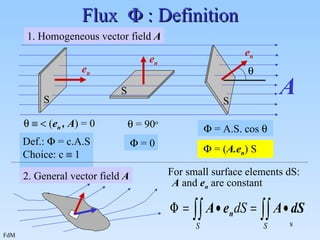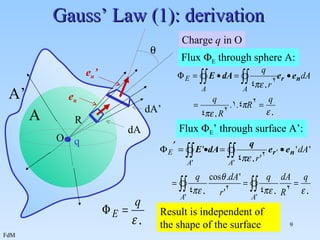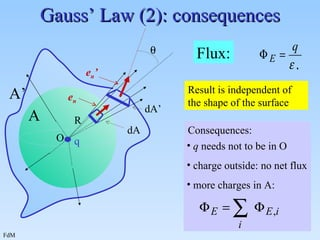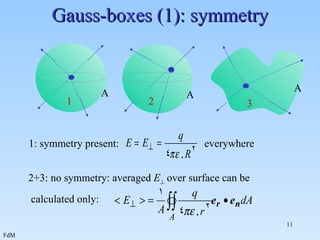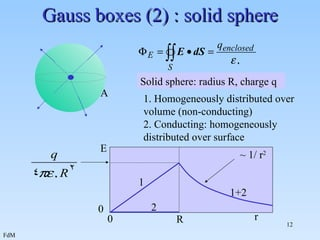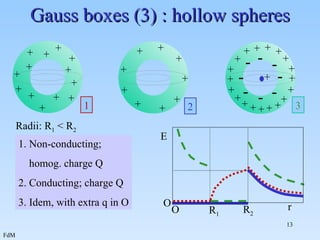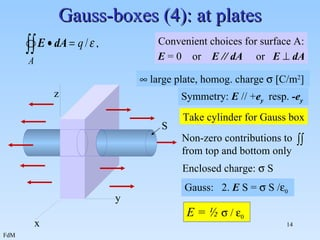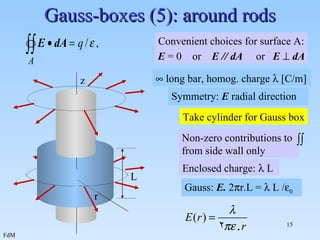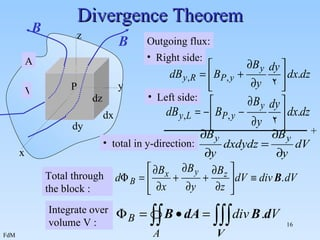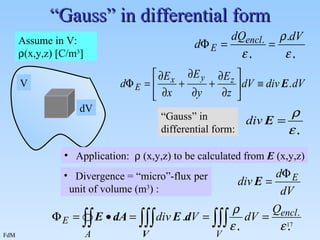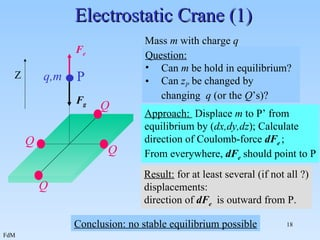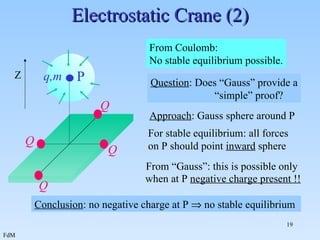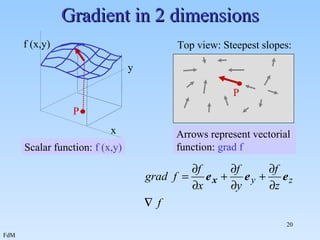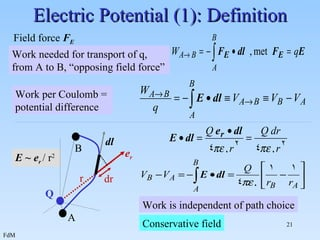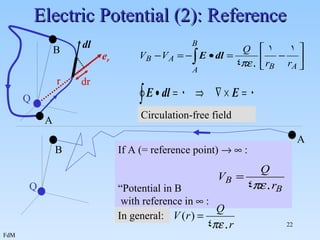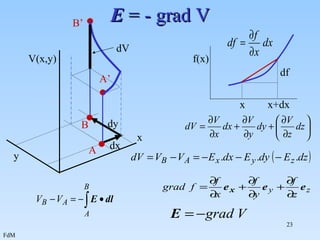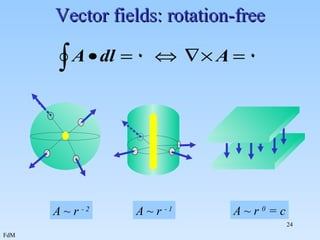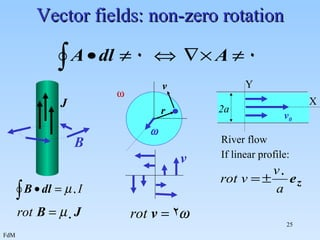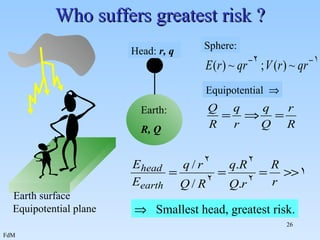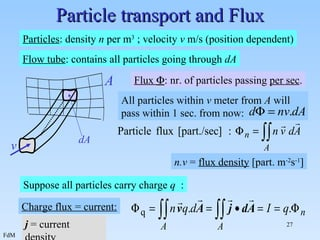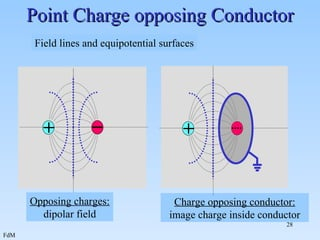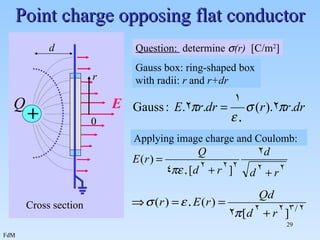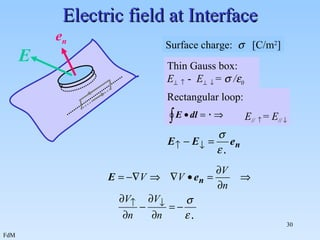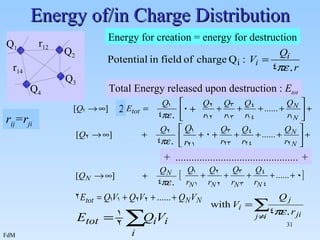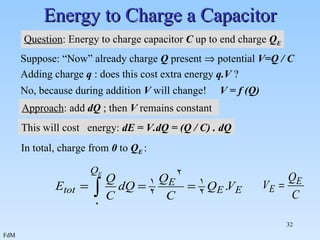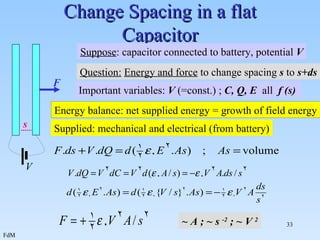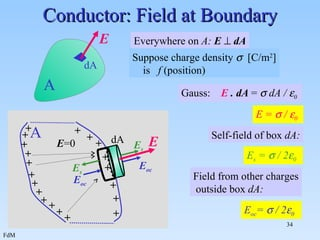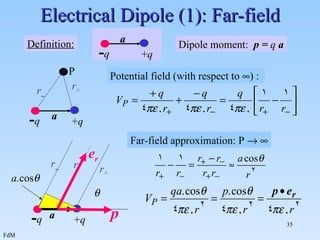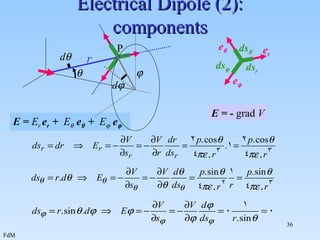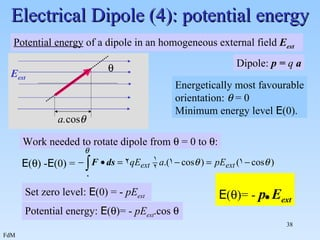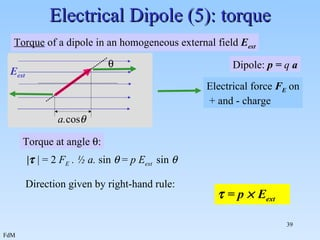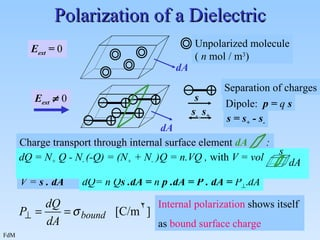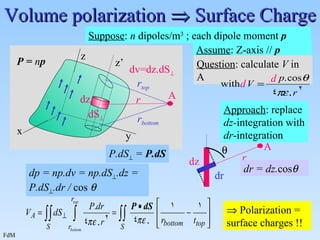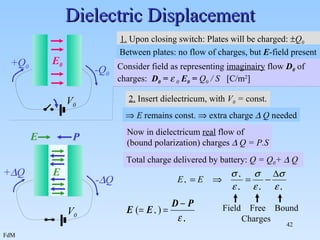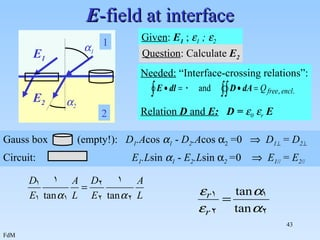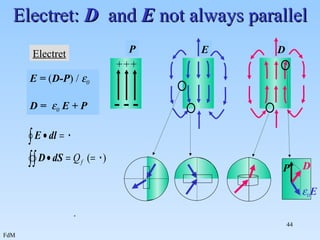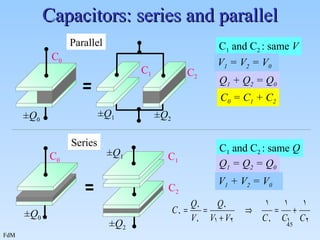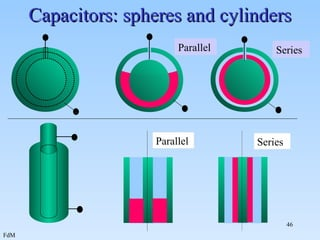1 von 46

### Electricity slides

• 1. Electromagnetism University of Twente Department Applied Physics First-year course on Part I: Electricity: Slides © F.F.M. de Mul
• 2.
• 3. Charge elements (1): rod, ring, disk Line Ring , radius R Disk , radius R  [C/m] O z dz dQ =  .dz  d  R  [C/m] dQ =  .R.d  P Symmetry: If  homogeneous: dQ =  .2  a.da  [C/m 2 ] d   a r P dE dQ =  .dA =  (a.d  ) da a  r ! 0 < a < R da
• 4. Charge elements (2): thin plate dQ =  dA =  dx.dy if plate  large : dE // e z P Thin plate ,  [C/m 2 ] x y z (1) z P dE r e r dE = dE x e x + dE y e y + dE z e z (2) (2) if  = f (x) only: x Use result for  long wire: z dE r e r P z P with d  =  . dx dE in XZ-plane = dA = dx.dy , at (x,y) (1)
• 5. Charge elements (3): tube and rod Avoid using r for radius !! Tube , radius R  [C/m 2 ] dz d  dA = R. d  . dz dQ =  R. d  . dz Cross section: thin ring, radius R dz d  Cross section: ring, radius a, width da Solid rod , radius R  [C/m 3 ] dV = (r.d  ).dr.dz dQ =  (r.d  ).dr.dz
• 6. Arc angle and Solid angle  r 1 radian [rad]  angle with arc length = radius r. 2  radians on circumference.  1 steradian [sr]  solid angle with surface area = r 2 . There are 4  sr on the surface. d  = (sin  .d  ) d   r sin  Solid angle:
• 7. Charge elements (4): on/in a sphere dA = u.v = (R.sin  .d  ).(Rd  ) 0 <  < 2  ; 0 <  <  dV = u.v.w = (r.sin  .d  ).(rd  ).dr 0 <  < 2  ; 0 <  <  0 < r < R R  d   d  R.sin  u v Spherical surface element r  d   d  r.sin  u v Spherical volume element w R
• 8. Flux  : Definition  = 0  = A.S. cos   = ( A.e n ) S e n e n e n A S S S  1. Homogeneous vector field A    ( e n , A ) = 0  = 90 o Def.:  = c.A.S Choice: c  1 2. General vector field A For small surface elements dS: A and e n are constant
• 9. Gauss’ Law (1): derivation Result is independent of the shape of the surface e n ’ dA’ Flux  E ’ through surface A’:  A’ R dA e n A Flux  E through sphere A: O q Charge q in O
• 10.
• 11. Gauss-boxes (1): symmetry 1: symmetry present: everywhere 2+3: no symmetry: averaged E  over surface can be calculated only: 1 2 3 A A A
• 12. Gauss boxes (2) : solid sphere 1. Homogeneously distributed over volume (non-conducting) 2. Conducting: homogeneously distributed over surface A Solid sphere: radius R, charge q 0 R r 0 E ~ 1/ r 2 1 2 1+2
• 13. Gauss boxes (3) : hollow spheres E R 1 R 2 r O O 2 3 1. Non-conducting; homog. charge Q 2. Conducting; charge Q 3. Idem, with extra q in O Radii: R 1 < R 2 + + + + + + + + + + + + + + + + + + + + + + + + + + + + + 1 + + + + + + + + + + + + + - - - - - - - -
• 14. Gauss-boxes (4): at plates Enclosed charge:  S Gauss: 2. E S =  S /  0 E = ½  /  0 Convenient choices for surface A: E = 0 or E // dA or E  dA  large plate, homog. charge  [C/m 2 ] x y z Symmetry: E // + e y resp. -e y S Take cylinder for Gauss box Non-zero contributions to from top and bottom only
• 15. Gauss-boxes (5): around rods Convenient choices for surface A: E = 0 or E // dA or E  dA Enclosed charge:  L Gauss: E. 2  r.L =  L /  0  long bar, homog. charge  [C/m] z Non-zero contributions to from side wall only Take cylinder for Gauss box L r Symmetry: E radial direction
• 16.
• 17.
• 18.
• 19. Electrostatic Crane (2) From Coulomb: No stable equilibrium possible. Question : Does “Gauss” provide a “ simple” proof? From “Gauss”: this is possible only when at P negative charge present !! Conclusion : no negative charge at P  no stable equilibrium Approach : Gauss sphere around P P q,m Q Q Q Q Z For stable equilibrium: all forces on P should point inward sphere
• 20. Gradient in 2 dimensions P Arrows represent vectorial function: grad f Top view: Steepest slopes: P x y f (x,y) Scalar function: f (x,y)
• 21. Electric Potential (1): Definition Field force F E Work needed for transport of q, from A to B, “opposing field force” Work per Coulomb = potential difference Work is independent of path choice Conservative field E ~ e r / r 2 dr e r r dl A B Q
• 22. Electric Potential (2): Reference Circulation-free field If A (= reference point)   : “ Potential in B with reference in  : In general: Q A B dr e r r dl Q A B
• 23. E = - grad V A B A’ B’ y x V(x,y) dx dV dy x x+dx df f(x)
• 24. Vector fields: rotation-free A ~ r 0 = c A ~ r - 1 A ~ r - 2
• 25. Vector fields: non-zero rotation 2a v 0 River flow If linear profile: X Y  r v  v J B
• 26. Who suffers greatest risk ?  Smallest head, greatest risk. Earth surface Equipotential plane Head: r, q Earth: R, Q Sphere: Equipotential 
• 27. Particle transport and Flux Particles : density n per m 3 ; velocity v m/s (position dependent) Flux  : nr. of particles passing per sec . All particles within v meter from A will pass within 1 sec. from now: n.v = flux density [part. m -2 s -1 ] Suppose all particles carry charge q : Charge flux = current: j = current density Flow tube : contains all particles going through dA dA A v
• 28. Point Charge opposing Conductor Opposing charges: dipolar field Field lines and equipotential surfaces Charge opposing conductor: image charge inside conductor + +
• 29. Point charge opposing flat conductor Question: determine  (r) [C/m 2 ] Applying image charge and Coulomb: + d Q Cross section E Gauss box: ring-shaped box with radii: r and r+dr r 0
• 30. Electric field at Interface Surface charge:  [C/m 2 ] Thin Gauss box: E   - E   =  /  0 E e n Rectangular loop: E //  = E // 
• 31. Energy of/in Charge Distribution Energy for creation = energy for destruction Total Energy released upon destruction : E tot + .............................................. + 2 Q 2 Q 3 Q 4 r 12 r 14 Q 1 r ij =r ji
• 32. Energy to Charge a Capacitor Question : Energy to charge capacitor C up to end charge Q E Suppose: “Now” already charge Q present  potential V=Q / C Adding charge q : does this cost extra energy q.V ? No, because during addition V will change! V = f (Q) Approach : add dQ ; then V remains constant This will cost energy: dE = V.dQ = (Q / C) . dQ In total, charge from 0 to Q E :
• 33. Change Spacing in a flat Capacitor Important variables: V (=const.) ; C, Q, E all f (s) Energy balance: net supplied energy = growth of field energy Supplied: mechanical and electrical (from battery) ~ A ; ~ s -2 ; ~ V 2 Question: Energy and force to change spacing s to s+ds F Suppose : capacitor connected to battery, potential V s V
• 34. Conductor: Field at Boundary Everywhere on A: E  dA Suppose charge density  [C/m 2 ] is f (position) Gauss: E . dA =  dA /  0 E =  /  0 Self-field of box dA: E s =  / 2  0 Field from other charges outside box dA: E oc =  / 2  0 A + + + + + + + + + + + + + + + + + + + + E =0 dA E E s E s E oc E oc A dA E
• 35. Electrical Dipole (1): Far-field Dipole moment: p = q a Potential field (with respect to  ) : Far-field approximation: P   - q + q a Definition: - q + q a r - r + P - q + q r - r + r  a. cos  a e r p
• 36. Electrical Dipole (2): components E = E r e r + E  e  + E  e  E = - grad V r   P d  d  e  e  e r ds r ds  ds 
• 37. Electrical Dipole (3): field lines E = E r e r + E  e  E r E   r e r e  Field lines perpendicular to equipotential planes
• 38. Electrical Dipole (4): potential energy Potential energy of a dipole in an homogeneous external field E ext Dipole: p = q a Energetically most favourable orientation:  = 0 Minimum energy level E (0). Work needed to rotate dipole from  = 0 to  : Set zero level: E (0) = - pE ext Potential energy: E (  )= - pE ext .cos  E (  )= - p . E ext  E ext a. cos  E (  ) - E (0) =
• 39. Electrical Dipole (5): torque Dipole: p = q a Torque at angle  :  = p  E ext |  | = 2 F E . ½ a. sin  = p E ext sin  Direction given by right-hand rule: Torque of a dipole in an homogeneous external field E ext a. cos   E ext Electrical force F E on + and - charge
• 40. Polarization of a Dielectric V = s . dA dQ= n Q s .dA = n p .dA = P . dA = P  . dA Internal polarization shows itself as bound surface charge dA Unpolarized molecule ( n mol / m 3 ) E ext = 0 E ext  0 s Separation of charges Dipole: p = q s s = s + - s - s + s - dA Charge transport through internal surface element dA : dQ = N + Q - N - (-Q) = (N + + N - )Q = n.VQ , with V = vol s dA
• 41. Volume polarization  Surface Charge dz Approach : replace dz- integration with dr -integration dp = np.dv = np.dS  .dz = P.dS  .dr / cos  P.dS  = P.dS  Polarization = surface charges !! z x y P = n p Suppose : n dipoles/m 3 ; each dipole moment p Assume : Z-axis // p A Question : calculate V in A dz A dr r  dr = dz. cos  r r top r bottom z’ dS  dv=dz.dS  d d
• 42. Dielectric Displacement Consider field as representing imaginairy flow D 0 of charges: D 0 =  0 E 0 = Q 0 / S [C/m 2 ] Total charge delivered by battery: Q = Q 0 +  Q V 0 +Q 0 -Q 0 1. Upon closing switch: Plates will be charged:  Q 0 E 0 Between plates: no flow of charges, but E -field present 2. Insert dielectricum, with V 0 = const. -  Q +  Q  E remains const.  extra charge  Q needed Now in dielectricum real flow of (bound polarization) charges  Q = P.S P V 0 E E Field Free Bound Charges
• 43. E -field at interface Given : E 1 ;  1 ;  2 Question : Calculate E 2 Needed: “Interface-crossing relations”: Relation D and E: D =  0  r E E 1 E 2  1  2 1 2 Gauss box (empty!): D 1 .A cos  1 - D 2 .A cos  2 =0  D 1  = D 2  Circuit: E 1 .L sin  1 - E 2 .L sin  2 = 0  E 1 // = E 2 //
• 44. Electret: D and E not always parallel . E D Electret P - - - +++ E = ( D-P ) /  0 D =  0 E + P P D  0 E
• 45. Capacitors: series and parallel C 1 and C 2 : same V C 1 and C 2 : same Q Q 1 + Q 2 = Q 0 V 1 + V 2 = V 0 V 1 = V 2 = V 0 Q 1 = Q 2 = Q 0 C 0 = C 1 + C 2 C 0 ± Q 0 C 0 ± Q 0 C 1 C 2 ± Q 2 ± Q 1 Series C 2 C 1 ± Q 2 ± Q 1 Parallel
• 46. Capacitors: spheres and cylinders Parallel Series Parallel Series